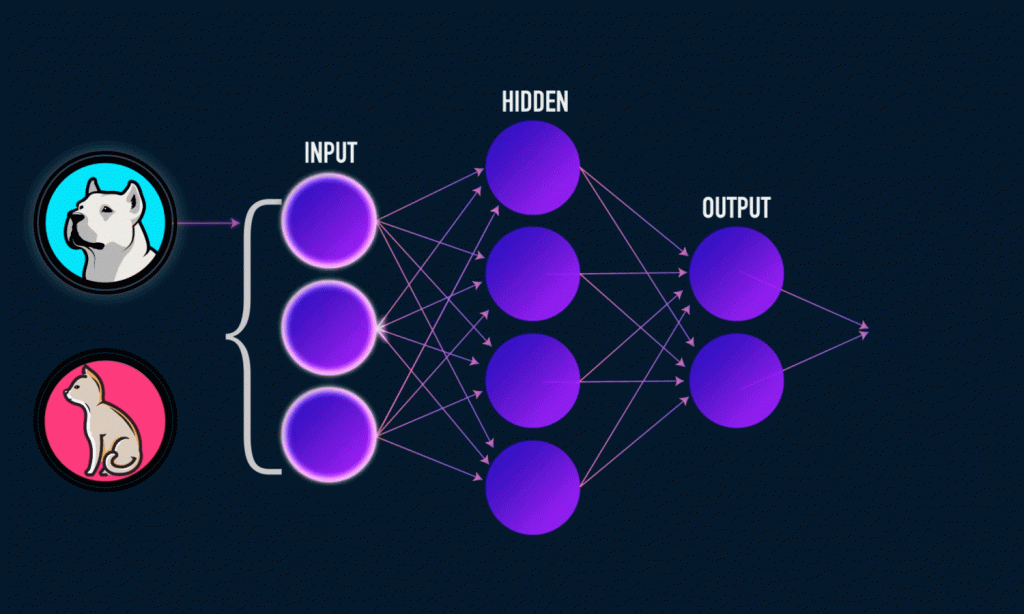# How have statistical machines influenced Machine Learning?The past few years have witnessed tremendous growth of machine learning across various industries. From being a technology of the future, machine learning is now providing resources for billion-dollar businesses. One of the latest trend observed in this field is the application of statistical mechanics to process complex information. The areas where statistical mechanics is applied ranges from natural models of learning to cryptosystems and error correcting codes. This article discusses how has statistical mechanics influenced machine learning.
What is Statistical Mechanics?
Statistical mechanics is a prominent subject of the modern day's physics. The fundamental study of any physical system with large numbers of degrees of freedom requires statistical mechanics. This approach makes use of probability theory, statistical methods and microscopic laws.
The statistical mechanics enables a better study of how macroscopic concepts such as temperature and pressure are related to the descriptions of the microscopic state which shifts around an average state. This helps us to connect the thermodynamic quantities such as heat capacity to the microscopic behavior. In classical thermodynamics, the only feasible option to do this is measure and tabulate all such quantities for each material.
Also, it can be used to study the systems that are in a non-equilibrium state. Statistical mechanics is often used for microscopically modeling the speed of irreversible processes. Chemical reactions or flows of particles and heat are examples of such processes.
So, How is it Influencing Machine Learning?
Anyone who has been following machine learning training would have heard about the backpropagation method used to train the neural networks. The main advantage of this method is the reduced loss functions and thereby improved accuracy. There is a relationship between the loss functions and many-dimensional space of the model's coefficients. So, it is very beneficent to make the analogy to another many-dimensional minimization problem, potential energy minimization of the many-body physical system.
A statistical mechanical technique, called simulated annealing is used to find the energy minimum of a theoretical model for a condensed matter system. It involves simulating the motion of particles according to the physical laws with the temperature reducing from a higher to lower temperature gradually. With proper scheduling of the temperature reduction, we can settle the system into the lowest energy basin. In complex systems, it is often found that achieving global minimum every time is not possible. However, a more accurate value than that of the standard gradient descent method can be found.
Because of the similarities between the neural network loss functions and many-particle potential energy functions, simulated annealing has also been found to be applicable for training the artificial neural networks. Other many techniques used for minimizing artificial neural networks also use such analogies to physics. So basically,  statistical mechanics and its techniques are being applied to improve machine learning, especially the deep learning algorithms.
If you find machine learning interesting and worth making a career out of it, join a machine learning course to know more about this. Also, in this time of data revolution, a machine learning certification can be very useful for your career prospects.

## drop us a message and keep in touch